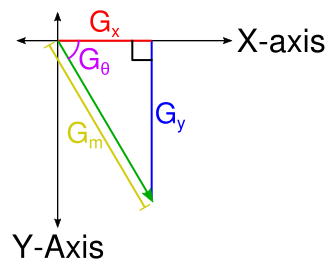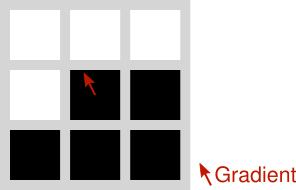This post is part of a series where I explain how to build an augmented reality Sudoku solver. All of the image processing and computer vision is done from scratch. See the first post for a complete list of topics.

The second part of the Canny algorithm involves finding the gradient at each pixel in the image. This gradient is just a vector describing the relative change in intensity between pixels. Since a vector has a magnitude and a direction, then this gradient describes how much the intensity is changing and in what direction.

The gradient is found using the Sobel operator. The Sobel operator uses two convolutions to measure the rate of change in intensity using surrounding pixels. If you studied Calculus, it’s worth noting that this is just a form of differentiation. One convolution measures the horizontal direction and the other measures the vertical direction. These two values together form the gradient vector. Below are the most common Sobel kernels. Unlike the Gaussian kernel, the Sobel kernels do not change so they can simply be hard coded.

K_x = [[-1,0,1],[-2,0,2],[-1,0,1]]

K_y = [[-1,-2,-1],[0,0,0],[1,2,1]]

Where K_x is the horizontal kernel and K_y is the vertical kernel.

A little more processing is needed to make the gradient usable for the Canny algorithm. If you’ve studied linear algebra or are familiar with polar coordinates the following should be familiar to you.First, the magnitude is needed which describes how quickly the intensity of the pixels are changing. By noting that the result of each convolution describes a change along the x- and y-axis, these two pairs put together form the adjacent and opposite sides of a right triangle. From there it should follow immediately that the magnitude is the length of the hypotenuse and can be solved using Pythagorean theorem.

G_m = sqrt(G_x^2 + G_y^2)

Where G_m is the magnitude, G_x is the result of the horizontal convolution, and G_y is the result of the vertical convolution.

The direction of the gradient in radians is also needed. Using a little trigonometry and the fact that we can calculate the tangent using the G_x and G_y components

tan(G_theta) = G_y / G_x

G_theta = arctan(G_y / G_x)

Where G_theta is the angle in radians. The arctan term requires special handling to prevent a divide by zero. Luckily, C++ (and most programming languages) have a convenient atan2 function that handles this case by conveniently returning +-0 or +-pi. The C++ variant of atan2 produces radians in the range [-pi,pi] with 0 pointing down the positive x-axis and the angle proceeding in a clockwise direction.

The following example demonstrates how to calculate the gradient for a region of pixels. A typical unsigned 8-bit data type is assumed with the black pixels having a value of 0 and the white pixels having a value of 255.P = [[255,255,255],[255,0,0],[0,0,0]]

G_x = P ** K_x = (255 * -1) + (255 * 0) + (255 * 1) + (255 * -2) + (0 * 0) + (0 * 2) + (0 * -1) + (0 * 0) + (0 * 1) = -510

G_y = P ** K_y = (255 * -1) + (255 * -2) + (255 * -1) + (255 * 0) + (0 * 0) + (0 * 0) + (0 * 1) + (0 * 2) + (0 * 1) = -1020

G_m = sqrt((-510)^2 + (-1020)^2) = 1140.39467

G_theta = arctan((-1020)/-510) = -2.03444 text{ radians} = 4.24888 text{ radians}

Here’s an implementation in code that, once again, ignores the border where the convolution would access out of bounds pixels.

Next is another step in the Canny algorithm which uses the gradient created here to build an image with lines where edges were detected in the original image.